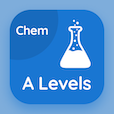Cambridge Online Courses (GCE)

A Level Chemistry Quizzes

A Level Chemistry Quiz PDF - Complete

# Number of Nucleons Multiple Choice Questions p. 188

Study Number of Nucleons multiple choice questions and answers, number of nucleons quiz answers PDF 188 to study A Level Chemistry course online. Atomic Structure and Theory MCQ trivia questions, number of nucleons Multiple Choice Questions (MCQ) for online college degrees. "Number of Nucleons MCQ" PDF eBook: melting points of group ii elements, oxidation numbers, electrolysis technique, solid state, number of nucleons test prep for accredited online college courses.

"Particular element posses the same number of electrons hence have the same" MCQ PDF: physical properties, chemical properties, density, and volume for ACT subject tests. Learn atomic structure and theory questions and answers to improve problem solving skills for ACT prep classes.

## Number of Nucleons Questions and Answers MCQs

MCQ: Particular element posses the same number of electrons hence have the same

chemical properties
physical properties
density
volume

MCQ: Ionic compounds only conduct electricity when they are in a state

solid
liquid
gas
molten

MCQ: Electrolysis is used to

produce metals
purify metals
refine metals
three of above

MCQ: The formula Na2O shows Na oxidation number to be

+1
+2
- 1
-2

MCQ: The lowest melting point among these elements is of

Be
Ca
Sr
Mg

### More Quizzes from A Level Chemistry Course